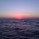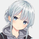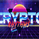จำนวนเข้าชม 1804
When designing filters it can be interesting to have information about their characteristics, which can be obtained from the set of filter coefficients (weights). The following script analyzes the impulse response of a filter in order to return the following information:

• Lag
• Smoothness via the Herfindahl index
• Percentage Overshoot
• Percentage Of Positive Weights

The script also attempts to determine the type of the analyzed filter, and will issue warnings when the filter shows signs of unwanted behavior.

DISPLAYED INFORMATION AND METHODS

The script displays one box on the chart containing two sections. The filter metrics section displays the following information:

- Lag : Measured in bars and calculated from the convolution between the filter's impulse response and a linearly increasing sequence of value 0,1,2,3.... This sequence resets when the impulse response crosses under/over 0.

- Herfindahl index : A measure of the filter's smoothness described by Valeriy Zakamulin. The Herfindahl index measures the concentration of the filter weights by summing the squared filter weights, with lower values suggesting a smoother filter. With normalized weights the minimum value of the Herfindahl index for low-pass filters is 1/N where N is the filter length.

- Percentage Overshoot : Defined as the maximum value of the filter step response, minus 1 multiplied by 100. Larger values suggest higher overshoots.

- Percentage Positive Weights : Percentage of filter weights greater than 0.

Each of these calculations is based on the filter's impulse response, with the impulse position controlled by the Impulse Position setting (its default is 1000). Make sure the number of inputs the filter uses is smaller than Impulse Position and that the number of bars on the chart is also greater than Impulse Position. In order for these metrics to be as accurate as possible, make sure the filter weights add up to 1 for low-pass and band-stop filters, and 0 for high-pass and band-pass filters.

The comments section displays information related to the type of filter analyzed. The detection algorithm is based on the metrics described above. The script can detect the following type of filters:

• All-Pass
• Low-Pass
• High-Pass
• Band-Pass
• Band-Stop

It is assumed that the user is analyzing one of these types of filters. The comments box also displays various warnings. For example, a warning will be displayed when a low-pass/band-stop filter has a non-unity pass-band, and another is displayed if the filter overshoot is considered too important.

HOW TO SET THE SCRIPT UP

In order to use this script, the user must first enter the filter settings in the section provided for this purpose in the top section of the script. The filter to be analyzed must then be entered into the:
`f(input)`
function, where `input` is the filter's input source. By default, this function is a simple moving average of period length. Be sure to remove it.

If, for example, we wanted to analyze a Blackman filter, we would enter the following:

```f(input)=>
pi = 3.14159,sum = 0.,sumw = 0.
for i = 0 to length-1
k = i/length
w = 0.42 - 0.5 * cos(2 * pi * k) + 0.08 * cos(4 * pi * k)
sumw := sumw + w
sum := sum + w*input[i]
sum/sumw```

EXAMPLES

In this section we will look at the information given by the script using various filters. The first filter we will showcase is the linearly weighted moving average ( WMA ) of period 9.

As we can see, its lag is 2.6667, which is indeed correct as the closed form of the lag of the WMA is equal to (period-1)/3, which for period 9 gives (9-1)/3 which is approximately equal to 2.6667. The WMA does not have overshoots, this is shown by the the percentage overshoot value being equal to 0%. Finally, the percentage of positive weights is 100%, as the WMA does not possess negative weights.

Lets now analyze the Hull moving average of period 9. This moving average aims to provide a low-lag response.

Here we can see how the lag is way lower than that of the WMA . We can also see that the Herfindahl index is higher which indicates the WMA is smoother than the HMA . In order to reduce lag the HMA use negative weights, here 55% (as there are 45% of positive ones). The use of negative weights creates overshoots, we can see with the percentage overshoot being 26.6667%.

The WMA and HMA are both low-pass filters. In both cases the script correctly detected this information. Let's now analyze a simple high-pass filter, calculated as follows:

`input - ‎sma(input,length)`

Most weights of a high-pass filters are negative, which is why the lag value is negative. This would suggest the indicator is able to predict future input values, which of course is not possible. In the case of high-pass filters, the Herfindahl index is greater than 0.5 and converges toward 1, with higher values of length. The comment box correctly detected the type of filter we were using.

Let's now test the script using the simple center of gravity bandpass filter calculated as follows:

`‎wma(input,length) - ‎sma(input,length)`

The script correctly detected the type of filter we are using. Another type of filter that the script can detect is band-stop filters. A simple band-stop filter can be made as follows:

`input - (‎wma(input,length) - ‎sma(input,length))`

The script correctly detect the type of filter. Like high-pass filters the Herfindahl index is greater than 0.5 and converges toward 1, with greater values of length. Finally the script can detect all-pass filters, which are filters that do not change the frequency content of the input.

The script can give warning when certain filter characteristics are detected. One of them is non-unity pass-band for low-pass filters. This warning comment is displayed when the weights of the filter do not add up to 1. As an example, let's use the following function as a filter:

`sum(input,length)`

Here the filter pass-band has non unity , and the sum of the weights is equal to length. Therefore the script would display the following comments:

We can also see how the metrics go wild (note that no filter type is detected, as the detected filter could be of the wrong type). The comment mentioning the detection of high overshoot appears when the percentage overshoot is greater than 50%. For example if we use the following filter:

`5*wma(input,length) - 4*sma(input,length)`

The script would display the following comment:

We can indeed see high overshoots from the filter:

@alexgrover for PineCoders
Tools and ideas for all Pine coders: http://www.pinecoders.com
Our Pine FAQ & Code: http://www.pinecoders.com/faq_and_code/
Get Pine news and tips on Telegram: https://t.me/PineCodersSquawkBox

## ความคิดเห็นbig thank you!
this is so timely @alexgrover as it will help test an MA concept i've been working on for some time - trying to get better responsiveness and less lag than HMA.
i'm getting lag of 1.15 and a 0.28 H. Index with 0% overshoots. so am I on the right track? i'm not a DSP expert..
ตอบกลับjust found out my question is silly, cause the lag will be a function of the length - maybe that's a possible improvement to this code ? to represent the lag as a % of the length - that would enable apple-to-apple comparison between different filters / MA types.
ตอบกลับ@RedKTrader, Its not a bad idea, but it would make the script less easier to use for other users. You can always tweak the script for your needs.
ตอบกลับalexgrover
@alexgrover, yep! got it..

see what you did now :) i had to test my MA against your script :)

here's how it looks (yellow) against an HMA (blue) and a WMA (purple) .. i found out my MA has less overshooting than HMA especially at relatively higher lengths - made me happy. thank you!

ตอบกลับPushing the envelope here, I love it!
ตอบกลับ